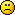# Square Root Formula

Jump to Last Post 1-6 of 6 discussions (10 posts)
1. Does anyone have a formula for finding the square root of a number? I know that one can use a calculator but there is a formula in the calculator in order for it to perform. I would like to have the formula along with an example.

Thank you.
Bernard Moses

1. Try the second of the two methods shown in this:

http://www.homeschoolmath.net/teaching/ … orithm.php

I must say the first method (the "guess and check" one) looks a lot easier!

2. Calculators have built-in routines which compute exponential functions and natural logarithms. With these tools in place they can quickly compute a multitude of goodies, such as the square root. A calculator does it as follows:

sqrt(X) = e (0.5 * ln (X))

where e = exponential func, and ln = natural log

That's how a slide rule does it as well.

But, there are hundreds of successive approximating algorithms that you can use to write your own programming routine to calculate square roots from scratch.

3. Hey Bernard,
I just posted a hub about a quicker way of finding the square root. See it here http://hubpages.com/hub/Shortcut-Method … uare-Roots

2. As far as I can recall the formula is obtained through calculus. I believe it is called Newton's Method for approximating roots of equations. (any root can be obtained.)
Or perhaps a Taylor Series is used.
When I was in High School (precalculator days) we learnt a method for computing square roots. This method can be found in older high school math books.
An interesting (and simpler method though a calculator is helpful for this) is to obtain the square root through successive approximations.
For instance you need to find the square root of 6.
6 lies between 4 and 9.Therefore the square root lies between 2 and 3. calculate (2.5)^2 This is 6.25 a tad too big. Now try (2.25)^2 Continue by changing the decimal value until you obtain the accuracy you need.

3. I asked my high school math teacher this and he couldn't answer! Crazy!

4. It would be hard for us to calculate square roots the same way that a calculator would.  The use an approach based on logarithms.

Math can be expressed mechanically by a cam or electronically through a circuit or by an algorithm like a computer or calculator.

Most estimation methods are iterative:

Lets look at a way to find square roots:

Well lets memorize five for now

2^1/2=1.41
3^1/2=1.73
5^1/2=2.24
7^1/2=2.65
11^1/2=3.32

If you notice, I picked only prime numbers because all other numbers are combination of the primes.  Now normally, I would memorize 20.  I used to do this and would very quickly calculate square roots.

Lets start here:  Okay we don't have 6.  6^1/22^1/2)(3^1/2)=2.44

Now you can see why I just use primes:

Now lets say we want to find 80^1/2.  This is (16^1/2)(5^1/2)=4(2.24)=8.96

Try your calculator and it will come incredibly close.

So lets say we want to find the square root of 331.

Pretty tricky right?

331 is close to 330 okay
and 330 is 2*5*3*11

and 330^1/22^1/2)(5^1/2)(3^1/2)(11^1/2)=1.41*2.24*1.73*3.32=18.14

331^1/2=18.19

You can actually iterate at this point and you will be very close.

If you have 20 primes memorized, you can calculate very large numbers fast and accurately.

I hope this helps.

1. I have to seperate the equals form the paranthesis because it gives a sad face.330^1/2= (2^1/2)(5^1/2)(3^1/2)(11^1/2)=1.41*2.24*1.73*3.32=18.14

6^1/2= (2^1/2)(3^1/2)=2.44

5.0
Deborah Sextonposted 8 years ago
6. Well, there is a way of calculating a square root by hand. Seeing how many people were having trouble with this I've just written a hub on 'Square Root of a Number by Hand'. And yes, it can be done by hand, calculators were programmed by people who knew the algorithm. Have fun ... always ... with Maths!

working